# Ultrasound

Ultrasound shows the impact that active experiments and feature gates are having on a metric of interest. This is a powerful tool for root causing unexpected changes in a metric.

Ultrasound presents a reverse perspective of the Pulse view. While Pulse measures the impact of a new feature on all your metrics, Ultrasound allows you to focus on a single metric and identify which tests are impacting it the most.

## How to read Ultrasound​

1. Navigate to the Ultrasound section on the Statsig console: https://console.statsig.com/
2. Select a metric that you want to observe from the selector drop down at the top of the page.
3. Select the time window that you want to observe.
4. The Feature Lifts panel shows two numbers. The number in parantheses is the absolute change in the metric driven by the users in the test group. The Delta % is percentage change relative to the topline value of the metric. Both numbers represent the daily average impact over the time window you have selected.

In the example below, the new_search_algo_v2 is driving an additional 65,070 add_to_cart events per day over the last 30 days. This is equivalent to a 5.98% average daily lift in this metric, which has oscillated bewtween 1M and 1.3M events per day during this time period.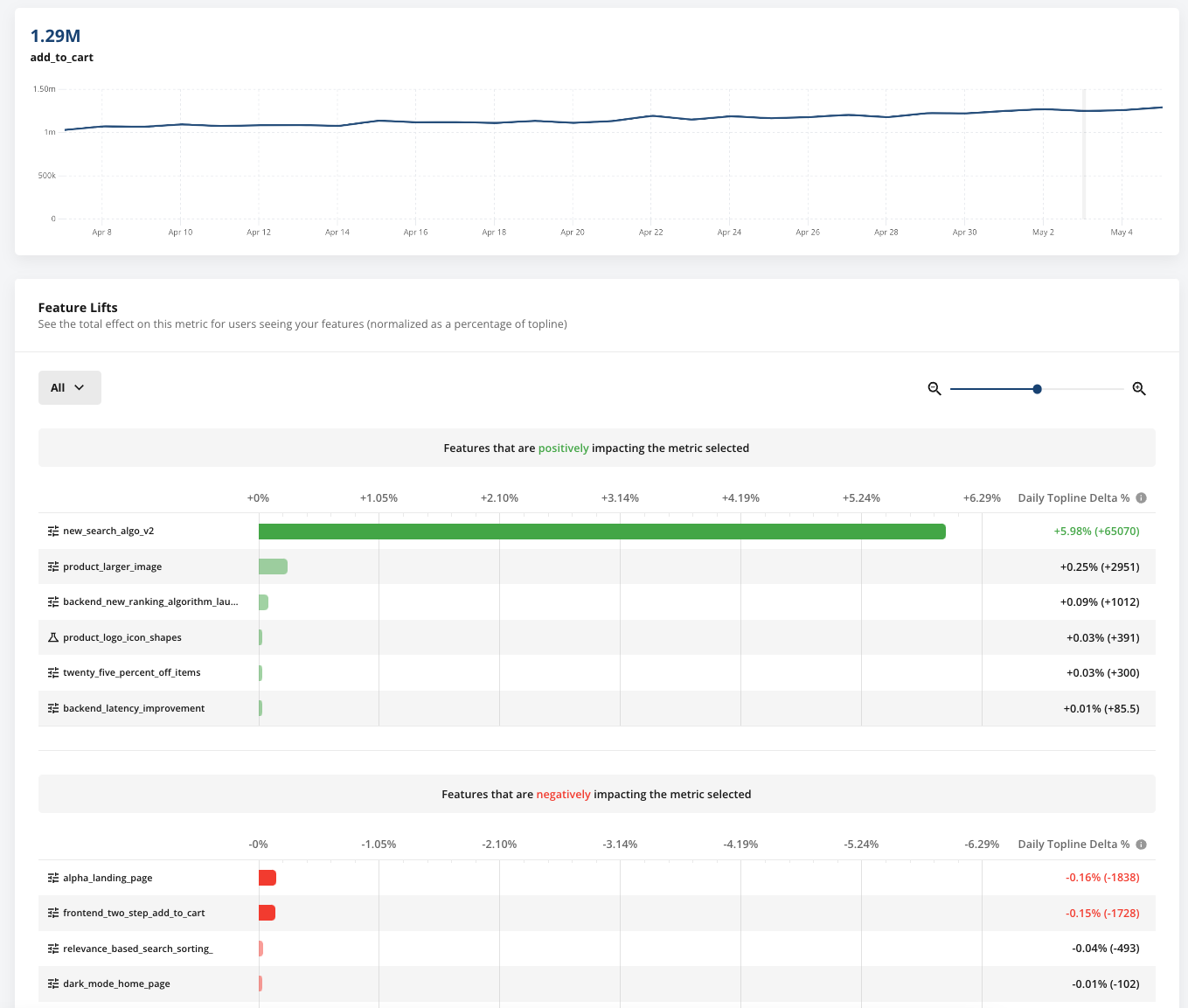## How lifts are calculated​

The impact of an active experiment on the overall topline metric depends on:

• The metric lifts caused by the experiment. This is the test vs. control comparison you see in Pulse.
• The number of users participating in the test group, which depends upon the targeting gate, layer allocation, and test group size. A large relative lift from a small experiment may have negligible impact on the topline metric.

The topline impact is computed daily and averaged over the selected date. The exact calculation depends on whether the metric represents an absolute quantity or a ratio.

Count and sum metrics (event_count, event_dau, sum)

The absolute impact is derived directly from the experiment results: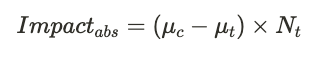where μt and μc represent the mean metric value for the test and control group, respectively, and Nt is the number of users in the test group.

Knowing the absolute impact and the overall metric value (as seen in the metrics dashboard), we can compute the relative impact: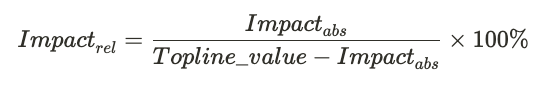Ratio and mean metrics To properly derive the topline impact on a ratio metric we must understand the impact on the numerator (X) and denominator (Y) separately: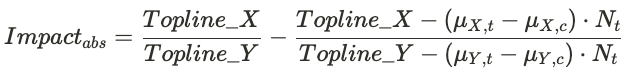where μX,t and μY,t represent the average numerator and denominator values for the test group, and similarly for the control group. Toplin_X and Topline_Y are the overall numerator and denominator values for the topline metric.

The relative impact for ratio metrics is obtained by dividing the absolute impact by the topline value of the metric that we would expect without this experiment: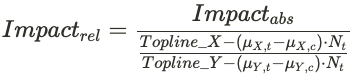Confidence intervals To determined whether the impact from a given experiment is statistically significant, we calculate the confidence intervals for each of the impact equations shown above. The variance is obtained using the Delta method. This properly accounts for the correlation between the various numerator and denominator terms and leverages Taylor expansion to linearize expressions containing non-linear combinations of experiment variables.